# Perimeter and areaPage 1

#### WATCH ALL SLIDES

Slide 1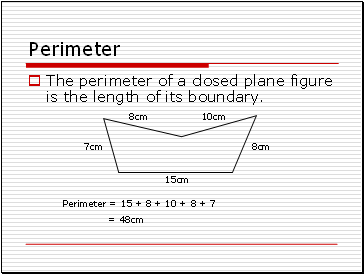## Perimeter

The perimeter of a closed plane figure is the length of its boundary.

15cm

10cm

8cm

7cm

8cm

Perimeter = 15 + 8 + 10 + 8 + 7

= 48cm

Slide 2Perimeter

Rectangle

Square

Triangles

P = 8 + 5 + 8 + 5

P = l + b + l + b

= 2(l + b)

L= 8cm

P = 6 + 6 + 6 + 6

P = l + l + l + l

= 4l or 4s

L=6cm

b=4cm

a

b

c

P = a + b + c

Slide 3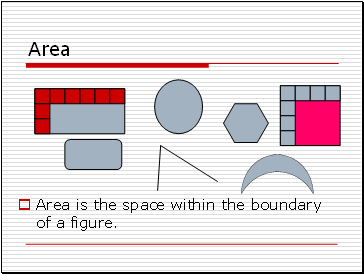## Area

Area is the space within the boundary of a figure.

Slide 4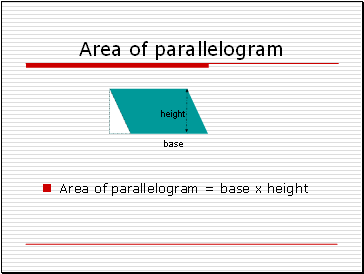## Area of parallelogram

Area of parallelogram = base x height

base

height

Slide 5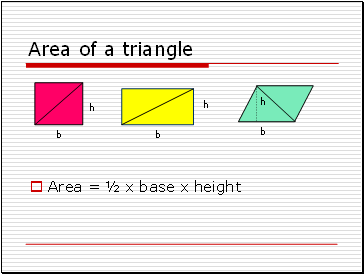## Area of a triangle

Area = ½ x base x height

b

b

h

h

h

b

Slide 6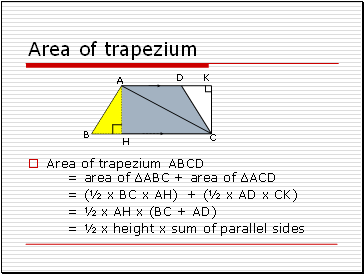## Area of trapezium

Area of trapezium ABCD = area of ΔABC + area of ΔACD

= (½ x BC x AH) + (½ x AD x CK)

= ½ x AH x (BC + AD)

= ½ x height x sum of parallel sides

A

D

C

H

B

K

Slide 7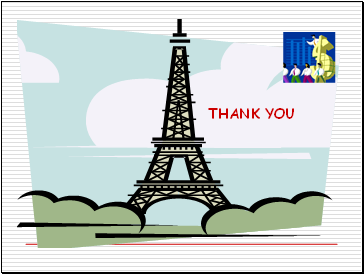THANK YOU